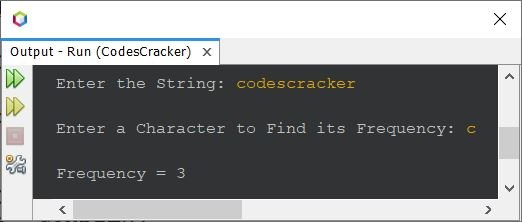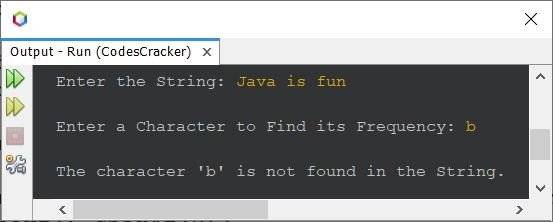# Java Program to Find Frequency of a Given Character in a String

This article is created to cover a program in Java that find and prints the frequency or the occurrences of a given character in a given string. For example, if given string is codescracker and character is c, then the output will be 3, because 'c' occurs 3 times in the string "codescracker".

The question is, write a Java program to find frequency of a character in a string. Both character and string must be received by user at run-time of the program. The program given below is the answer to this question:

```import java.util.Scanner;

public class CodesCracker
{
public static void main(String[] args)
{
String str;
char ch, strCh;
int strLen, i, count=0;
Scanner s = new Scanner(System.in);

System.out.print("Enter the String: ");
str = s.nextLine();
System.out.print("\nEnter a Character to Find its Frequency: ");
ch = s.next().charAt(0);

strLen = str.length();
for(i=0; i<strLen; i++)
{
strCh = str.charAt(i);
if(ch==strCh)
count++;
}

System.out.println("\nFrequency = " +count);
}
}```

Here is its sample run with user input codescracker as string and c as character to find its frequency:The above program can also be created/written in this way:

```import java.util.Scanner;

public class CodesCracker
{
public static void main(String[] args)
{
int strLen, i, count=0;
Scanner s = new Scanner(System.in);

System.out.print("Enter the String: ");
String str = s.nextLine();
System.out.print("\nEnter a Character to Find its Frequency: ");
char ch = s.next().charAt(0);

for(i=0; i<str.length(); i++)
{
if(ch==str.charAt(i))
count++;
}

if(count==0)
else
System.out.println("\nThe Frequency of character \'" +ch+ "\' = " +count);
}
}```

Here is its sample run with user input codescracker dot com as string and c as character to find its frequency or occurrences:Here is another sample run with user input Java is fun as string and b as character:Java Online Test

« Previous Program Next Program »